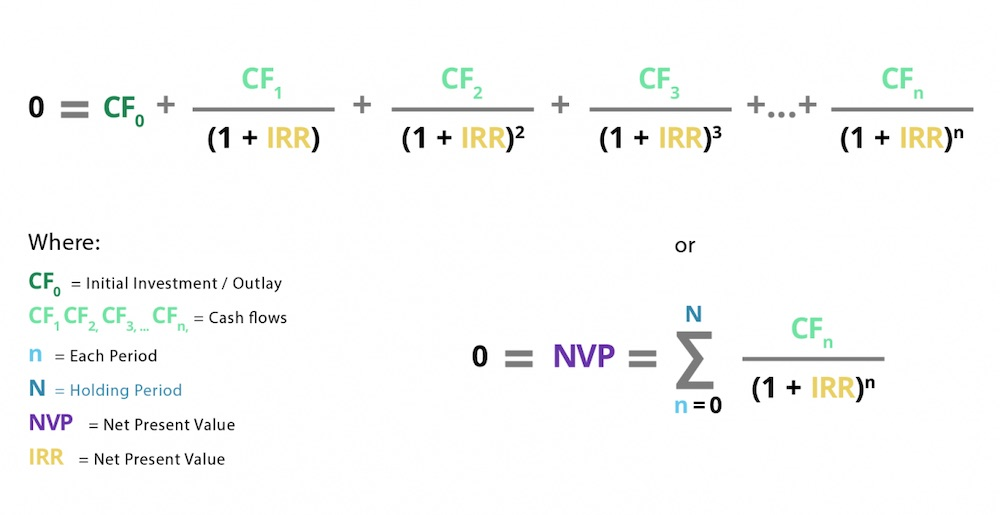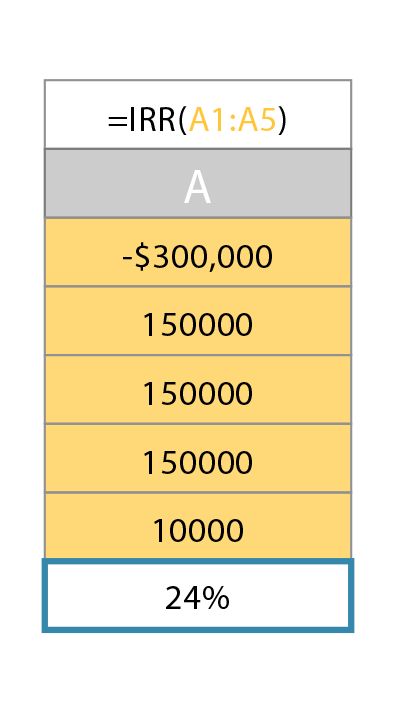# Internal Rate of Return | IRR

Updated June 6, 2021

## What Is Internal Rate of Return (IRR)?

Internal rate of return (IRR) is the discount rate that makes the net present value of all cash flows (both positive and negative) equal to zero for a specific project or investment.

IRR may also be referred to as the discounted cash flow rate of return (DCFROR).

### What Does IRR Tell You About a Project?

The internal rate of return is used to evaluate projects or investments. The IRR estimates a project’s breakeven discount rate (or rate of return) which indicates the project’s potential for profitability.

Based on IRR, a company will decide to either accept or reject a project. If the IRR of a new project exceeds a company’s required rate of return, that project will most likely be accepted. If IRR falls below the required rate of return, the project should be rejected.

## What Is a Good IRR?

A positive IRR means that a project or investment is expected to return some value to the organization.

A negative IRR, however, can happen mathematically if the project’s cash flows are alternately positive and negative over its expected duration. A negative IRR is indicative of a more complicated cash flow stream that may make the metric less useful.

### Be Careful of Making Comparisons

Nailing down a specific “good” IRR metric is impossible unless you know the industry for that investment, as well as the company’s cost of capital.

For example, a good IRR in real estate is generally 18% or above, but maybe a real estate investment has an IRR of 20%. If the company’s cost of capital is 22%, then the investment won’t add value to the company. The IRR is always compared to the cost of capital, as well as to industry averages. In order to know what a “good” IRR is you need to know more about the investment opportunity.

## Internal Rate of Return Formula

The IRR formula is as follows:

0 (NPV) = P0 + P1/(1+IRR) + P2/(1+IRR)2 + P3/(1+IRR)3 + . . . +Pn/(1+IRR)n

Where:

• P0 equals the initial investment (cash outflow)

• P1, P2, P3..., equals the cash flows in periods 1, 2, 3, etc.

• IRR equals the project's internal rate of return

• NPV equals the Net Present Value

• N equals the holding periods

OR## IRR Calculation Example in Excel

A general rule of thumb is that the IRR value cannot be derived algebraically. Instead, IRR must be found by mathematical trial-and-error. While it may seem difficult to learn how to calculate IRR manually, Excel automatically performs this function.

### 1. Initial Cash Flow into the Spreadsheet

Keep in mind that this initial investment has to be a negative number. For this example, type -300,000 into the A1 cell of the spreadsheet.

### 2.  Subsequent Cash Flow Values for Each Period

In the cells directly under the initial investment amount, type cash flow values. For example, if your initial cash flow is in cell A1, type the following cash flows into cell A2, A3, A4, etc.

### 3. Instruct Excel to Calculate the IRR

To instruct Excel to calculate IRR, type in the function command "=IRR(A1:A4)" into the A5 cell (directly under all the values).  When you hit the Enter key, the IRR value should be displayed in that cell.This same procedure can be followed for any data set, as long as the cash flow values are listed one after another in a column, directly under the initial investment amount.

If you would like to use a business calculator, check out these step-by-step IRR calculation examples.

## Internal Rate of Return Solved Examples

You can also use IRR to calculate expected returns on investments or projects.

### IRR Example #1: How IRR and NPV Are Related

To gain a better understanding the IRR formula, start with the net present value formula and a simple short-term project (then expand).

Let’s say Company X has a year-long project that is going to cost \$1,000 and has a discount rate of 8%. At the end of the year, the company will receive \$1,300. Calculating the NPV for this project looks like this:

NPV=-1,000 + 1,3001.08=203.70

In general, if the NPV is greater than 0, a project is worth pursuing.

The IRR calculation for this same project puts the NPV at 0. When the NPV is 0, it acts as the break-even point. If that’s the case, it will look like this:

0=-1,000 + 1,300(1+IRR)

Notice how the discount rate of 8% is replaced with IRR, but the formula remains the same.

Solving for IRR, you will get 0.30 or 30%.

#### What Does This Mean for the Project?

Company X can’t forget about their discount rate of 8% (also known as the opportunity cost), used to calculate the NPV. IRR is compared to the opportunity cost to make a decision for accepting or declining a project.  As a general rule, if the IRR is higher than the opportunity cost, a company can accept the project or investment. If the project’s “breakeven” return is greater than the company’s opportunity cost, the company could take on this project and increase its value.

### IRR Example #2: Discover the Yield of an Investment

Let’s look at IRR from the perspective of a large investment over a period of 3 years:

Let’s say Company Y must decide if they should purchase a piece of factory equipment for \$300,000. The equipment would only last three years, but it is expected to generate \$150,000 of additional annual profit during those years.

Company Y also thinks it can sell the equipment for scrap afterward for about \$10,000. Using IRR, Company Y can determine whether the equipment purchase is a better use of its cash than its other investment options, which should return about 10%.

Here is how the IRR equation looks in this scenario:

0 (NPV) = -\$300,000 + (\$150,000)/(1+0.2431) + (\$150,000)/(1+0.2431)2 + (\$150,000)/(1+0.2431)3 + \$10,000/(1+0.2431)4

The investment's IRR is 24.31%, which is the rate that makes the net present value equal to zero.

#### What Does This Mean for the Investment?

In this case, Company Y is comparing other investment opportunities of 10%. The equipment would have to have an opportunity cost of more than 24.31% in order to lose value for the Company, which is much higher than the 10% opportunity cost. It would be prudent for the company to buy the equipment.

## Limitations of Internal Rate of Return

IRR allows managers to rank projects by their overall rates of return rather than their net present values. The investment or project with the highest IRR is usually preferred. This easy comparison makes IRR attractive, but it does come with limitations:

• IRR works only for investments that have an initial cash outflow (the purchase of the investment) followed by one or more cash inflows. IRR can't be used if the investment generates interim negative cash flows.

• IRR does not measure the absolute size of the investment or the return. This means that IRR can favor investments with high rates of return, even if the dollar amount of the return is very small.

• For example, a \$1 investment returning \$3 will have a higher IRR than a \$1 million investment returning \$2 million. The latter, however, brings in \$1 million dollars (instead of just \$2).

Overall, the IRR method is best suited for analyzing venture capital and private equity investments. These typically have multiple cash investments and a single cash outflow at the end via IPO or sale.

## What's the Difference Between IRR and WACC?

The weighted average cost of capital (WACC) is how much it costs for a company to finance itself using capital from bondholders, other lenders, and shareholders. In relation to the IRR formula, WACC is the "required rate of return" that a project or investment's IRR must exceed to add value to the company. This return rate may also be referred to as a hurdle rate, opportunity cost, or cost of capital.

### IRR vs. WACC Example

For example, if a company's WACC is 10%, proposed projects must have an IRR of 10% or higher to add value to the company. If a proposed project yields an IRR lower than 10%, the company's cost of capital is more than the expected return from the proposed project or investment.

Here's another way to look at it: If you used your credit card with a 10% annual interest rate (like the WACC) to buy a lemonade stand, you'd need the lemonade stand to return more than 10% every year (similar to the IRR) to earn a profit. Otherwise, you'd be losing money every year and wouldn't add value to your net worth.

## The Difference Between NPV and IRR

Net present value (NPV) measures how much value (in dollars) a project or investment could add. By contrast, IRR projects the rate of return that a project or investment can generate.

Both NPV and IRR can help provide analysts with a clearer picture of projects (or investments) that can add the most value to an organization.

### Example: IRR vs. NPV

Let’s look back at the example from earlier:

The \$300,000 machine would return \$460,000 in additional profits (\$150,000 + \$150,000 + \$150,000 + \$10,000 = \$460,000).

Assuming a 5% discount rate and running the numbers through an NPV calculator the result is a net present value of \$116,714.23.

That NPV figure gives a dollar amount of value, providing more information to make a better-informed decision.

## Key Takeaways for IRR

Typically, the higher the IRR, the higher the rate of return a company can expect from a project or investment. Therefore, IRR can be an incredibly important measure of a proposed investment’s success.

However, a capital budgeting decision must also look at the value added by the project. Decision-makers usually look at both the IRR and NPV in making capital budgeting decisions.

All of our content is verified for accuracy by Rachel Siegel, CFA and our team of certified financial experts. We pride ourselves on quality, research, and transparency, and we value your feedback. Below you'll find answers to some of the most common reader questions about Internal Rate of Return | IRR.

## Is a Negative IRR Possible?

Yes, a negative IRR is possible. A negative IRR would mean that the total cash flows after investment are less than the initial investment. Put another way, the undiscounted cash flows add up to less than the initial value.

## Is NPV Better Than IRR?

One metric is not necessarily better than the other as both serve a purpose. That said, the NPV is more realistic when evaluating certain projects. This is especially true for projects with non-normal cash flows or long-term projects where the discount rate may change over time. NPV will always provide an easy “accept or reject” answer for analysts. That said, using one metric to analyze a project is not recommended and would simplify the analysis. If IRR can be reviewed it should be.

Rachel Siegel, CFA - 141Rachel Siegel, CFACFA Charterholder

Chartered Financial Analyst

Rachel Siegel, CFA is one of the nation's leading experts at ensuring the accuracy of financial and economic text.  Her prestigious background includes over 10 years creating professional financial certification exams and another 20 years of college-level teaching.